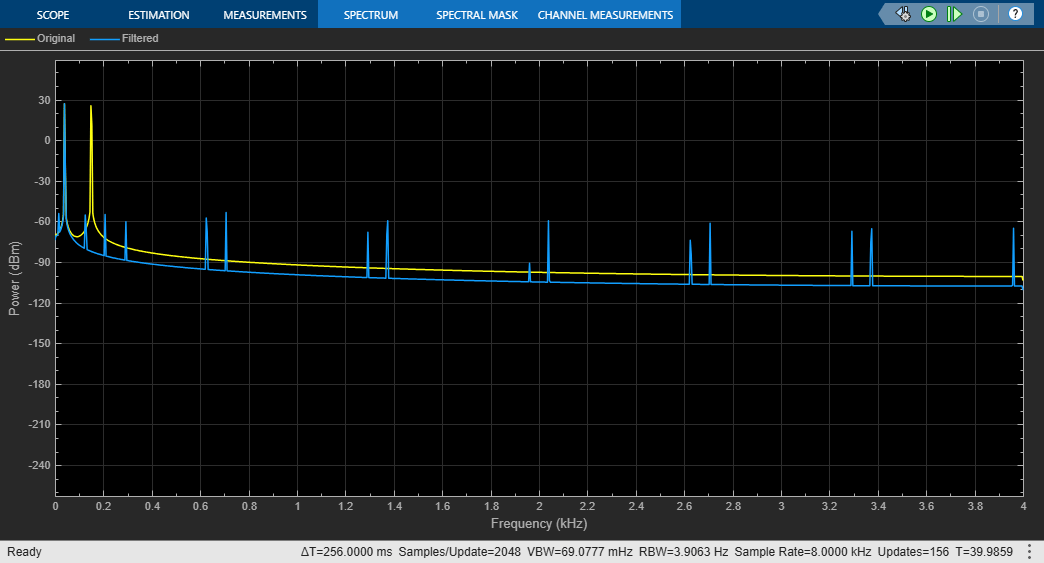# Two-Stage Multirate Narrow Lowpass Filter

Implement a two-stage multirate narrow lowpass filter using the FIR Decimation and FIR Interpolation blocks.

Open the model.The input signal contains a sum of two sine waves with frequencies 40 Hz and 100 Hz, respectively. The sample rate of the signal is 8000 Hz. The input sinusoidal signal is filtered using a two-stage multirate narrow lowpass filter. The filter is implemented as a subsystem block containing a two-stage decimation filter followed by a two-stage interpolation filter. The decimation filter contains two FIR Decimation blocks: first block with a decimation factor of 12 and second block with a decimation factor of 4. The interpolation filter similarly contains two FIR Interpolation blocks: first block with an interpolation factor of 4 and second block with an interpolation factor of 12. The overall filter you design is a narrow lowpass filter with a passband frequency of 75 Hz.

Run the model. Use a Spectrum Analyzer to compare the power spectra of the original and filtered signals. The block uses the `Filter Bank` method for spectral estimation. For more details, see Spectrum Estimation — Filter Bank. The 40 Hz frequency falls in the passband of the filter and is unattenuated. The 100 Hz frequency falls in the stopband of the filter and is therefore attenuated.